• 在很多问题里，通常要找到一个系数a，使得给定的误差函数值形如f(d(x,a)-t)期望最少，其中t为真实值，d(x,a)是关于自变量x，系数为a的函数(通常是线性函数)(注：x和a通常是向量，表示... 对于平方误差函数E = Σ(d(x,a

在很多问题里，通常要找到一个系数a，使得给定的误差函数值形如f(d(x,a)-t)期望最少，其中t为真实值，d(x,a)是关于自变量x，系数为a的函数(通常是线性函数)(注：x和a通常是向量，表示一组自变量和对应的一组系数)。给定的误差函数可以是误差的绝对值期望，也可以是误差值的平方的期望。通常平方比绝对值更常用，因为方便做微分运算。
对于平方误差函数E = Σ(d(x,a) – t(x) )2，它的最小值称为最小均方误差(MMSE)，我们经常要求函数d(x,a)中系数a，使得误差最小，从而做出判断。例如在信源最优量化中，需要寻找一个最优量化值，用来代表附近范围的值，使得误差最小。在视频运动估计，预测编码等应用中，找出最好的预测值，使得与真实值最接近。在曲线拟合中，需要估计出一条曲线方程，使得样本点集与曲线上的估计值的均方误差最小，这种最小平方拟合也译作最小二乘法。(又是高斯这大牛搞出来的)
为求局部极小值，对误差方程求导，使导数为0，可得到关于系数向量a线性方程组，解出线性方程组便得到的解为最优的系数，参见：
http://www.tyut.edu.cn/kecheng/jisff/dzja/ch6/ch6-1.htm

http://mathworld.wolfram.com/MethodofSteepestDescent.html
以一维情况为例，我们首先估计一个初始系数值a0, 然后求出误差函数的在该点导数f'(x), 迭代直到第r步系数值ar, 使该点梯度绝对值小于某一精度阀值。
ar = ar-1 – k f'(x) | ar-1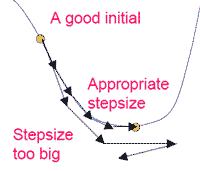其中k为速度常数，取过大会使在极值点附近振荡，过小则迭代次数太多。
对于多维的情况，则利用Jacobi矩阵计算偏导数。
更复杂的方法有共轭梯度法，牛顿-拉夫逊(Newton-Raphson, N-R法)， Levenberg-Marquardt方法

关于共轭梯度法求函数最小值的方法，有一篇很好的指导文章：

CMU计算机系写的共轭梯度法解惑，非常详细地讨论了梯度下降和共轭梯度法，还有一些线性代数的复习。

关于Levenberg- Marquardt的C＋＋程序：
http://www.ics.forth.gr/~lourakis/levmar/

关于他的原理：
http://www.cs.toronto.edu/~roweis/notes/lm.pdf


展开全文c
• 探索平方误差期望值内涵 一、条件期望 　条件期望在概率论与统计中也被称为条件数学期望，它的用途主要是用于实际的预测性问题。如对于两个互有影响的随机变量，如果我们知道其中一个随机变量X=a这一观测值，...
文章向导

条件期望
最小二乘法
探索平方误差的期望值内涵

一、条件期望
条件期望在概率论与统计中也被称为条件数学期望，它的用途主要是用于实际的预测性问题。如对于两个互有影响的随机变量，如果我们知道其中一个随机变量X=a这一观测值，要据此去估计或预测随机变量Y的取值。
首先，想到的自然是选择条件概率P(Y=b|X=a)值最大时的b作为答案，如果需要尽可能地提高估计的精度，那么此方法无疑是很合理的。
另一种做法做法则是求在X=a时Y的条件分布，并计算出相应的期望值，即：
$E\left( Y|X=a \right) \equiv \,\,\sum_b{b}P\left( Y=b|X=a \right) （1-1）$
上式也就是条件期望的定义式。但需要注意到，对于取值不同的X，其条件期望E(Y|X=a)的值也不同。所以，如果能知道X各种取值出现的概率，那么条件期望的最终计算结果则与一般的期望值E(Y)一致，即：
$E\left( Y \right) =\sum_a{E\left( Y|X=a \right) P\left( X=a \right)} （1-2）$
现在来详细证明式(1-2)是如何得出的，先将式(1-1)代入进行推导。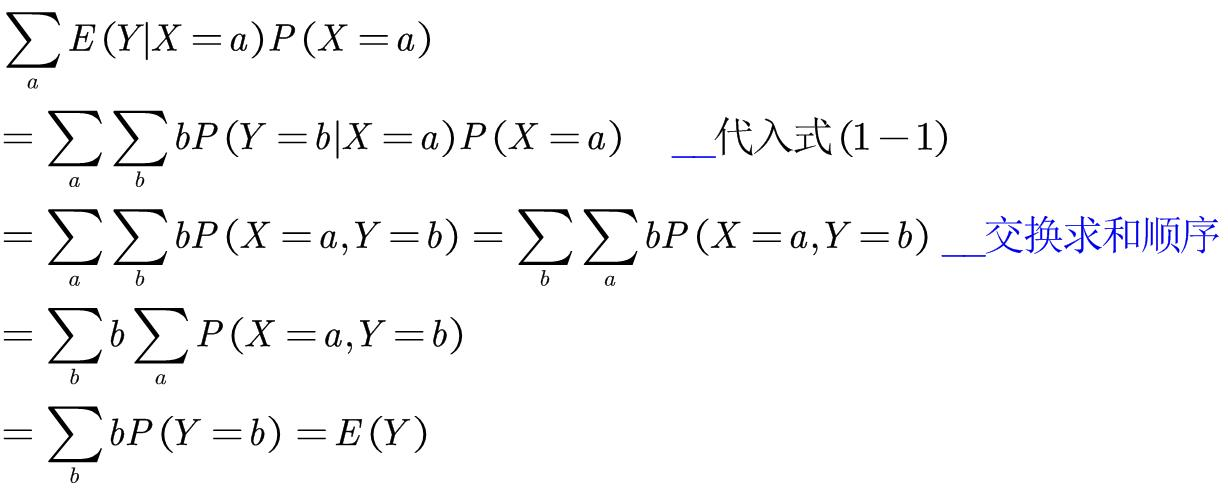二、最小二乘法
最小二乘法又称最小平方法，是数学中一种常用的优化方法，即通过最小误差的平方和寻找数据的最佳函数匹配。

1.实例推导
接下来这部分，则是与条件期望相关的一个应用实例。我们先思考如下问题，假设有条件分布$P(Y=b|X=a)$，试设计一个程序，如何使得在输入X之后输出Y的估计值$\hat{Y}$。并使平方误差$\left( Y-\hat{Y} \right) ^2$的期望值$E\left[ \left( Y-\hat{Y} \right) ^2 \right]$ 尽可能小。
乍一看问题貌似很复杂，实际上要求的就是输入X后输出Y的估计值函数中，使$E\left[ \left( Y-\hat{Y} \right) ^2 \right]$ 的值最小时所对应的那个$\hat{Y}=g(X)=E(Y|X=x)$。
再具体一点，其实问题的答案就是之前所谈及的条件期望g(a)=E(Y|X=a)。这点也符合人们的直观理解，估计值$\hat{Y}$与Y十分接近时，平方误差自然小。
为了简化问题的分析，可将X的取值范围给固定为{1,2,3}，此时平方误差的期望值如下所示。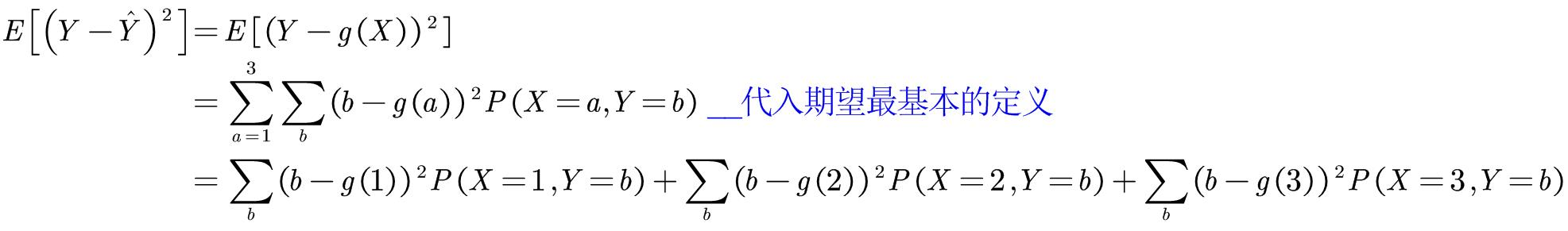上图中最后一行等式可分为3个部分，取决于g(1)的量+即取决于g(2)的量+即取决于g(3)的量。那么，现在的问题就转化为求各部分的解，然后则能得出最佳的g。即定义g(1)，使$\sum_b{\left( b-g\left( 1 \right) \right) ^2P\left( X=\text{1,}Y=b \right)}$有最小值，同理g(2)和g(3)类似。
接着，根据上述的思路来找出这样的g(1)，为表示方便用$g_1$替代g(1)。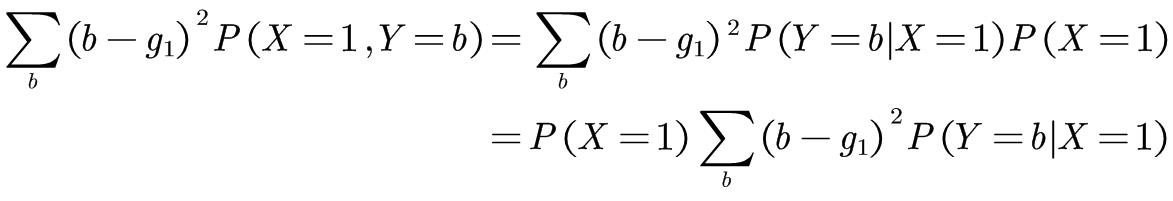求该式的最小值等价于求$h_1(g_1)=\sum_b{\left( b-g_1 \right) ^2P\left( Y=b|X=1 \right)}$的最小值。好，马上就要成功了，让我们来计算它的微分。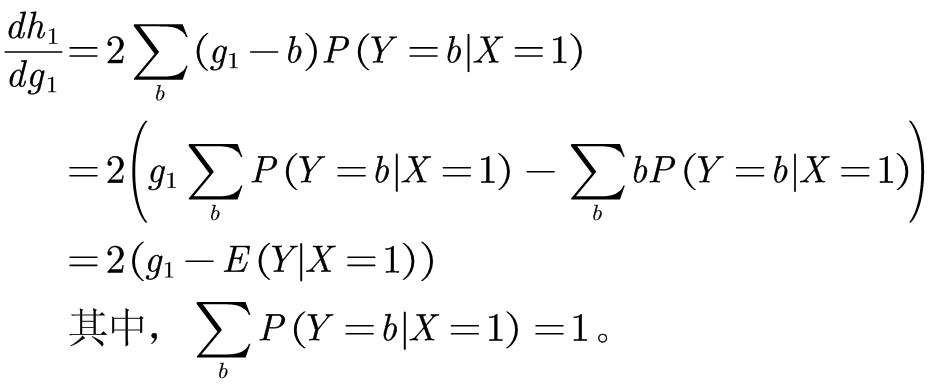由极值的判定关系可知，当$dh_1/dg_1=0$时，即$g_1=E(Y|X=1)$时，$h_1(g_1)$能取到最小值，$h_2(g_2)$、$h_3(g_3)$同理可得。最后，从而推得$g(a)=E(Y|X=a)$的结论。

2.如何理解所求得的g(a)？
从$g(a)=E(Y|X=a)$形式上来看，它就是一个普通的函数。只要提供一个具体的数值a，它就会返回一个确定的值g(a)。那么，如果给g提供一个随机变量X，就能得到一个与X对应的随机变量$\hat{Y}=g(X)=E(Y|X=x)$。好吧，表达式看起来依然是那么的抽象。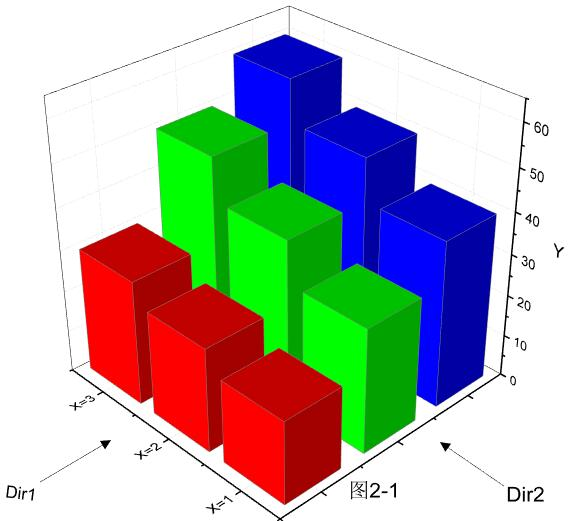不妨看看图2-1，X=1,2,3分别对应着前面所提及的三个部分，可以把这三个部分想象为各自独立的平行世界，每个平行世界的Y值(柱状体的高)不尽相同(Dir2方向观察)，且同一平行世界下的Y值也不等(Dir1方向观察)。可能有些读者会迷惑，为啥同一平行世界下的Y值也不相同，那么请思考下条件分布P(Y|X=1)。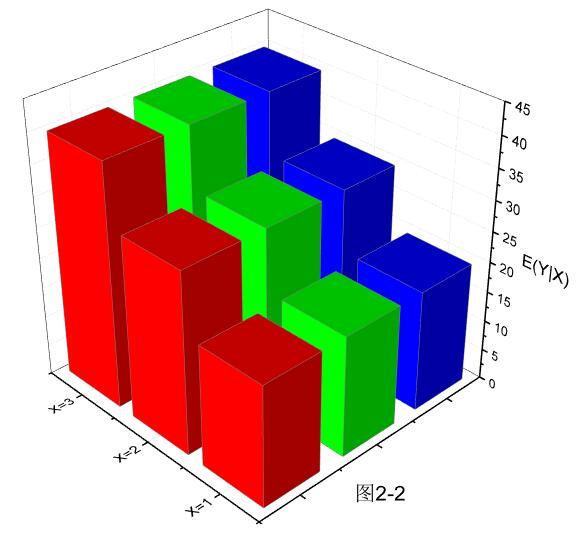接着看图2-2，此时柱状体的高为E(Y|X)的值，而且有趣的是同一平行世界下的高现在是相等的。这点很好理解，因为求的是期望，那么最终结果肯定是将同一X区域下的不同高度给统一起来(也就是平均效果)。若是将三个平行世界的结果再继续综合起来，则最终得到E(Y)。

三、探索平方误差的期望值内涵
1. 从偏差的平方到方差
谈及平方误差，读者的第一反应或许会是方差。那么，让我们先从方差开始谈起。设随机变量X的数学期望E(X)=μ现在我们需要计算它的实际取值x与$\mu$的差距。$|x-\mu |$可能是最为直观的方式，但落实到具体的计算时，绝对值的存在往往会带来许多不便(如分类讨论、曲线折角处不可微等)。于是，人们通常用偏差的平方$\left( x-\mu \right) ^2$来描述问题。
这样的描述也非常符合离散程度的定义，因为仅当$X=\mu$时，误差为0，其余情况误差总是存在且大于0。目前离方差的定义：
$V\left[ X \right] =E\left[ \left( X-\mu \right) ^2 \right]$
很接近了，但还差一个取期望。Ok，思考下为何还要取一个期望才能得到方差？首先，$\left( X-\mu \right) ^2$得到的是一个随机值，而我们希望得到的是一种数值固定的指标，故取其期望来消除其中的随机性。
2.平方误差的期望值
正式往下说之前，读者应该先了解这个公式$V\left[ X \right] =E\left( X^2 \right) -E\left( X \right) ^2$。
试证：对于常量a，当$E\left( X \right) =\mu \text{，}V\left( X \right) =\sigma ^2$时，有等式$E\left[ \left( X-a \right) ^2 \right] =\left( \mu -a \right) ^2+\sigma ^2$成立。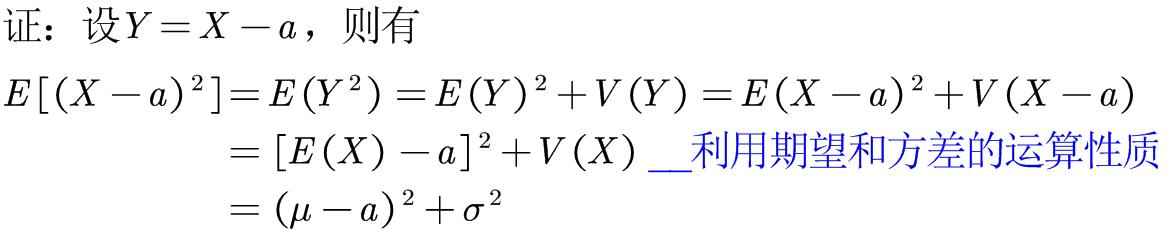证明完毕，现在来说道说道如何理解这个等式。假设某工厂要生产尺寸恰好为a cm的零件，而最终实际产品的尺寸为X cm。那么，现在$\left( X-a \right) ^2$就为平方误差。与上述证明的等式相比较，可发现该误差被分解为如下两种误差：(期望值的平方误差)+方差 =（由偏移引起的误差）+（由离散引起的误差）。
更为专业的说法则是，系统误差(又称偏性误差，数值整体偏移)与随机误差(又称机会误差，数值离散)。
那么，由于生产工艺的不同，最终得到的产品在两种误差上的表现也会不同。如系统误差较小，随机误差较大。虽然看似误差较小，但其实数值X较为离散。

参阅资料
程序员的数学<概率统计>
概率论与数理统计<浙大版>
普林斯顿微积分读本


展开全文• MMSE:Minimum Mean Squared Error,思想是将预测值和真值的误差平方期望（均值）最小化，以使得预测值尽量逼近真值，和真值差距尽可能小，这个理论指导下，我们来推导最优帧内预测的加权系数向量。 帧内预测是...
最小均方误差（MMSE）意义下的最优帧内预测推导：

MMSE:Minimum Mean Squared Error,思想是将预测值和真值的误差的平方的期望（均值）最小化，以使得预测值尽量逼近真值，和真值差距尽可能小，这个理论指导下，我们来推导最优帧内预测的加权系数向量。

帧内预测是用于消除单帧图像内部的空间冗余的图像压缩方法，视频压缩中I帧(关键帧)就只需要帧内预测编码。

先把所有像素值去中心化，即减去均值再除以标准差，对得到的标准像素进行编码即可。则标准像素是标准高斯分布的，均值为0，方差为1。
这里帧内预测用已编码像素
$X_n=\{X_i\}^T,i=0,1,2\ldots ,K$
的线性加权和来预测待编码像素$x_j$，即
$\hat{x}_j=\sum_{i=0}^Kx_iw_i=X_n^TW_j$
所以关键，核心就是求解最优的预测权重向量$W_j=\{w_i\}^T,i=0,1,2\ldots ,K$。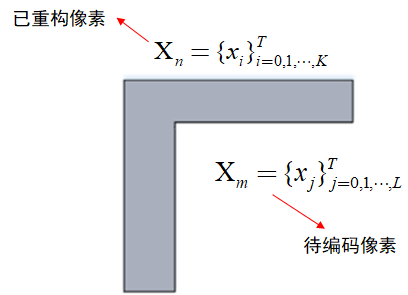之前不懂为甚么示意图画为这个形状，看了后面的例子就明白了，这是由于图像中像素的相关性与空间位置有关。
下面从均方误差开始推导：
$MSE=E[(x_j-{\hat{x}_j})^2]$
$=E[(x_j-\hat{x}_j)^T(x_j-\hat{x}_j)]$
$=E[(x_j-\sum_{i=0}^Kx_iw_i)^T(x_j-\sum_{i=0}^Kx_iw_i)]$
$=E[(x_j-X_n^TW_j)^T(x_j-X_n^TW_j)]$
$=E(x_j^2)-E(x_jX_n^TW_j)-E(x_jW_j^TX_n)+E(W_j^TX_nX_n^TW_j)$
注意其中$x_j, \hat{x}_j$ 都是scalar，转置值同。
为了求最优的权重向量$W_j$,将上面推导出来的MSE误差对$W_j$求导，令为0即可：
（标量对向量求导）
$\frac{\partial MSE}{\partial W_j}=\frac{\partial E(x_j^2)-E(x_jX_n^TW_j)-E(x_jW_j^TX_n)+E(W_j^TX_nX_n^TW_j)}{\partial W_j}$
$=0-E(x_jX_n)-E(x_jX_n)+E(X_nX_n^TW_j)+E(X_nX_n^TW_j)=0$
$E(x_jX_n)=E(X_nX_n^T)W_j$
$W_j=E(X_nX_n^T)^{-1}E(x_jX_n)$
其中$E(x_jX_n)$是待编码像素和已编码像素的互相关向量
$E(X_nX_n^T)$是已编码像素的自相关矩阵
所以根据推导，在最小均方误差意义下的最优帧内预测的线性加权系数向量就是已编码像素向量的自相关矩阵的逆矩阵作用于待编码像素和已编码像素的互相关向量。
需要注意的是，其中涉及到的标量对向量求导，用的是分母布局，即求导结果和分母维度一致。

下面展示一个帧内预测的例子：
假设图像内像素符合一阶Markov分布，即每个像素取值只与自己距离1个像素的3个neighbors有关，这里采用典型的3近邻结构，水平距离1个pixel的两个像素互相关系数为$\rho_h$,垂直距离1个pixel的两个像素互相关系数为$\rho_v$,以此类推得到水平距离p个像素，垂直距离q个像素的两点的互相关系数为$R(p,q)=\rho_h^p\rho_v^q$，可见距离越远，互相关系数越小（$0\leq\rho_v\leq1,0\leq\rho_h\leq1$）。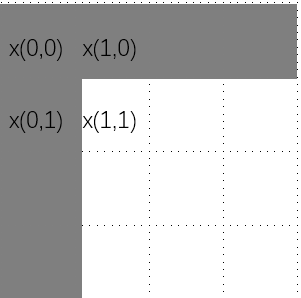如上图，灰色区域的像素已经编码，白色区域则可以直接根据灰色区域预测，无需再编码，因此可节省码率，这就是3近邻结构，x(1,1)像素的值仅由x(0,0),x(0,1),x(1,0)决定。将这三个近邻作为$X_n$,则自相关矩阵$R=E(X_nX_n^T)=$
$\begin{bmatrix} 1 & \rho_h & \rho_v \\ \rho_h & 1 & \rho_v\rho_h \\ \rho_v & \rho_v\rho_h &1 \\ \end{bmatrix}$
x(1,1)和三个近邻的互相关向量为$\begin{bmatrix} \rho_v\rho_h \\ \rho_v \\ \rho_h\end{bmatrix}$
所以在这种情况下最优的预测编码权重系数向量$W_j=R^{-1}\begin{bmatrix} \rho_v\rho_h \\ \rho_v \\ \rho_h\end{bmatrix}$


展开全文图像压缩 帧内预测
• 常见的损失函数有平方误差损失函数，交叉熵损失函数等，在分类任务中一般使用交叉熵损失函数，回归问题中一般使用平方误差损失函数。 损失函数的核心思想是建立一种预测结果和真实结果之间误差的衡量标准，而机器...
机器学习任务几乎没有例外的都使用了损失函数cost function这一概念。常见的损失函数有平方误差损失函数，交叉熵损失函数等，在分类任务中一般使用交叉熵损失函数，回归问题中一般使用平方误差损失函数。

损失函数的核心思想是建立一种预测结果和真实结果之间误差的衡量标准，而机器学习的优化目标就是让这个衡量项越小越好，这种差别也叫做风险，风险分为经验风险和结构风险两种，经验风险是样本集合的预测值和真实值的差的平方的期望，这个期望也叫期望风险，但是由于期望风险难以求解，常常以经验风险来代替，但是经验风险存在一定问题，说明在下面，所以才提出了结构风险，结构风险到提出者也是SVM的提出者，所以对于SVM的优化问题是围绕着模型的结构风险最小化进行的，结构风险包含了经验风险和正则化项两项，并要求它们同时达到最小化。

上文提到经验风险最小化存在问题是什么问题呢？这个问题就是当在训练集上得到很好的效果的时候，在测试集上往往得到很差的效果。这是因为模型在训练集上产生了过拟合，直观理解是最小二乘法（最小二乘也是机器学习包括深度学习的思想的一个很重要的来源），当存在一系列杂乱无章的数据的时候，我们先去拿直线来拟合它，结果发现效果很差，然后使用二次曲线，发现结果要好一点，于是我们认为加大曲线的阶次可以提升模型的拟合效果，我们可能终于找到了一个很高阶的曲线拟合训练数据得到了一个很好的效果，但是这个曲线用到测试数据上也很好吗？答案往往是否定的。这个时候采用的办法就是正则化的办法，正则化分为L0，L1，L2三种分别表示非零项的个数，各项绝对值之和，各项平方和，（虽然在几何上三种正则化都有自身的解释，但是在我看来，它们无一例外的都是用来提高特征向量的稀疏性，从而避免过拟合的）。其中L2范数最难理解但是也最好解释，直观上，一条高次曲线，减小高次项系数与低次项系数的比值可以使得这条高次曲线更加的缓和，缓和自然也就降低了过拟合。所以，在经验风险的后面加上一个正则化项或者叫稀疏项就可以得到既准确拟合又不至于过拟合的模型。拿这个模型用在测试数据上也会得到差不多的性能。

参考文献：

http://blog.csdn.net/shenxiaoming77/article/details/51614601

https://blog.csdn.net/github_34777264/article/details/78750340#commentBox

展开全文• 1、假设一对关系是某线性关系，且实际测量数据与该理想关系的偏差是高斯分布。那么一个n个测量值的集合，取n...利用最小二乘法可以简便地求得未知的数据，并使得这些求得的数据与实际数据之间误差平方和为最小。最...
• 一，均方误差 个人理解：平均的平方误差值，即，误差的...最小均方误差估计就是指估计参数时要使得估计出来的模型和真实值之间的误差平方期望最小。 三、协方差 个人理解：协，协同的（两个变量）之间的误差 .矩阵 线性代数
• 最小二乘(Least Square)准则：以误差平方最小作为最佳准则的误差准则 定义式中, ξ(n)是误差信号的平方和；ej是j时刻的误差信号， dj是j时刻的期望信号，Xj是j时刻的输入信号构成的向量， W表示滤波器的权...
• 一 线性回归（最小二乘法） 假设我们有n个样本数据，每个数据有p个特征值，然后p个特征值是线性关系。...误差平方的计算公式 Xi为行向量，A为列向量。 最小二乘法的目标就是取得最小的e对应的A，...机器学习算法
• 平方期望值.误差就是估计值与被估计量的差. 均方差满足等式 其中 也就是说，偏差是估计函数的期望值与那个无法观察的参数的差。 下边是一个具体例子.假设 即是一组来自正态分布的样本.　常...
• ## 最小二乘法与岭回归的介绍与对比

万次阅读 多人点赞 2017-11-11 00:20:20
一 线性回归（最小二乘法） 假设我们有n个样本数据，每个数据有p个特征值，然后p个特征值是线性关系。...误差平方的计算公式 Xi为行向量，A为列向量。 最小二乘法的目标就是取得最小的e对应的A，...线性回归 机器学习 LASSO
• 一、最小二乘法（线性回归） 假设我们有n个样本数据，每个数据有p个特征值，然后p个特征值是线性关系。 即对应的线性模型 ...误差平方的计算公式为： e=∑i=1n(yi−XiA)2e=∑i=1n(yi−XiA)2e=\...
• 通过经典成本函数，平方误差成本函数和“命中或遗漏”函数已经得出了各种估计量。 但是，绝对误差函数的关注较少。 在本文中，我们考虑基于频域中统计量和贝叶斯成本函数的幅度平方频谱（MSS）动机估计器，用于语音...研究论文
• lms算法跟Rosenblatt感知器相比，主要区别就是权值修正方法不一样。lms采用的是批量修正算法，Rosenblatt感知器使用的是单样本修正算法。...均方误差：样本预测输出值与实际输出值之差平方期望值，记为MES ...
• FILS设计了一种线性相位FIR滤波器，它可以最小化理想分段线性函数与滤波器在一组期望频带上的幅值响应之间的加权积分平方误差。 b = firls(n,f,a) b = firls(n,f,a,w) b = firls(n,f,a,'ftype') b = firls(n,f,a,w,'...matlab C
• Python实现最小均方算法(LMS)lms算法跟Rosenblatt感知器相比，主要区别就是权值修正方法不一样。lms采用的是批量修正... 均方误差：样本预测输出值与实际输出值之差平方期望值，记为MES 设:observed 为样本真值,pr算法
• lms算法跟Rosenblatt感知器相比，主要区别就是权值修正方法不一样。lms采用的是批量修正算法，Rosenblatt感知器使用的 是单样本修正算法。... 均方误差：样本预测输出值与实际输出值之差平方期望值，记为MES ...
• '''算法：最小均方算法(lms)均方误差：样本预测输出值与实际输出值之差平方期望值，记为MES设:observed 为样本真值,predicted为样本预测值,则计算公式:(转换为容易书写的方式，非数学标准写法,因为数学符号在这里...
• lms算法跟Rosenblatt感知器相比，主要...详细代码及说明如下：'''算法：最小均方算法(lms)均方误差：样本预测输出值与实际输出值之差平方期望值，记为MES设:observed为样本真值,predicted为样本预测值,则计算公式:...
• ## 克里金插值

万次阅读 多人点赞 2019-04-29 15:58:40
注：常用的估计准则 无偏估计：即假设状态的估计值与真实值的平均值相等。 最小二乘估计：不考虑数据的统计特性，如期望，方差等，直接用最小...简单来说就是，满足误差方差最小必满足误差平方最小，反之不成...
• 1.估计准则 常用的估计准则包括： ...简单来说就是，满足误差方差最小必满足误差平方最小，反之不成立。而无偏估计是最基本假设。 原文链接：https://blog.csdn.net/qinruiyan/article/details/5卡尔曼滤波 LS
• 接着集成算法讲讲GBDT和Xgboost，二者的原理其实差不多的，他们都属于提升算法。...损失函数若是回归则常取最小平方误差和绝对值误差；如果是回归则采用类似Logister的似然函数，属于指数损失函数。首先从常函数...机器学习 集成算法
• 【引言】 所有机器学习算法都旨在最小化或最大化目标函数，其中，将目标函数最小化的过程称为损失函数。 损失函数：是衡量预测模型预测期望...1、最小平方误差法（MSE） 2、平均绝对值误差法（MAE） 3、平滑平均绝...
• 把统计看了一遍就是为了这里! 线性回归假设函数为 y=θTXy=θTXy=\theta^TX 之前是根据函数图像推导出损失函数...假设误差服从正态分布，误差最小也就是期望为0。ϵϵ\epsilon~N(0,σ2σ2\sigma^2) 最大似然估计...
• 最小二乘法是解决线性、非线性拟合问题的一般方法, 基于最朴素的假设(无偏估计、最小误差)推导得到, 通常表现为代价函数的形式, 即统计样本的误差平方和(或均). 对于一个估计问题, 由于噪声的存在, 我们希望得到一...